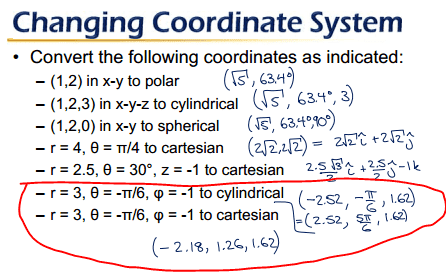# Cylindrical and Spherical Coordinates Changing

• theBEAST
So in this case, the angle would be something like -1.5*(π/6, π/6). Lastly, when ρ is negative does that mean the angle starts from the -z axis? Because when ρ is positive it starts from the positive z axis.

## Homework Statement

Convert the following as indicated:

1. r = 3, θ = -π/6, φ = -1 to cylindrical

2. r = 3, θ = -π/6, φ = -1 to cartesian

## The Attempt at a Solution

I just want to check if my answers are correct.

1. (2.52, -π/6, 1.62)
2. (-2.18, -1.26, 1.62)

theBEAST said:

## Homework Statement

Convert the following as indicated:

1. r = 3, θ = -π/6, φ = -1 to cylindrical

2. r = 3, θ = -π/6, φ = -1 to cartesian

## The Attempt at a Solution

I just want to check if my answers are correct.

1. (2.52, -π/6, 1.62)
2. (-2.18, -1.26, 1.62)

What coordinate system are these given in? ##r## is usually used in cylindrical coordinates and ##\rho## for spherical. Also, if ##\phi## is the spherical coordinate angle from the ##z## axis, it is usually restricted to the interval ##[0,\pi]##. Are you sure you copied the ##\phi## values correctly?

Mathematicians and physicists use ##\theta## and ##\phi## differently. You need to tell us which convention you're using here.

Here is the question with the answer key:In this case r = ρ and I'm not sure why phi is negative.

I don't think the answer key is correct.

The answer key is correct. You need to show us your calculations. To answer #1, it's probably most straightforward if you do #2 first and the convert from Cartesian to cylindrical.

vela said:
The answer key is correct. You need to show us your calculations. To answer #1, it's probably most straightforward if you do #2 first and the convert from Cartesian to cylindrical.

For number one, how can r be negative? They have -2.52 whereas I have 2.52. It is why I thought the answer key was wrong.

When r is negative, you reflect through the origin from where you'd otherwise be. In polar coordinates, for instance, the point r=-1, θ=π/4 would correspond to (-1/√2, -1/√2), which is where you'd end up if you reflected r=1, θ=π/4 through (0,0).

You'll notice they gave you a second answer where r is positive, but the angle has been changed to account for the reflection.

vela said:
When r is negative, you reflect through the origin from where you'd otherwise be. In polar coordinates, for instance, the point r=-1, θ=π/4 would correspond to (-1/√2, -1/√2), which is where you'd end up if you reflected r=1, θ=π/4 through (0,0).

You'll notice they gave you a second answer where r is positive, but the angle has been changed to account for the reflection.

Lastly, when ρ is negative does that mean the angle starts from the -z axis? Because when ρ is positive it starts from the positive z axis.

Not exactly. Reflection in spherical coordinates takes ##\phi \to \pi-\phi## and ##\theta \to \theta+\pi##. The change to ##\phi## effectively means you're measuring from the -z-axis, but you also have to accompany it with a rotation by 180 degrees about the z-axis.